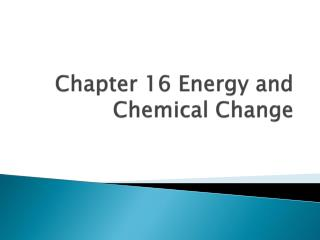DownloadDownload PresentationChapter 16 Energy and Chemical Change

# Chapter 16 Energy and Chemical Change

Download Presentation## Chapter 16 Energy and Chemical Change

- - - - - - - - - - - - - - - - - - - - - - - - - - - E N D - - - - - - - - - - - - - - - - - - - - - - - - - - -
##### Presentation Transcript

1. Chapter 16 Energy and Chemical Change

2. Nature of energy • Energy – the ability to do work or produce heat • Kinetic energy – energy of motion • Potential energy – stored energy • Chemical potential energy – stored energy because of composition

3. Measuring heat • Heat – energy process of flowing from a warmer object to a cooler object • Calorie – the amount of heat required to raise the temperature one degree Celsius • Joule – SI unit of heat and energy

4. Calories • When your body breaks down sugars and fats to form carbon dioxide and water, these exothermic reactions generate heat that can be measured in Calories

5. Specific Heat • Specific heat – amount of heat required to raise the temperature of one gram of that substance by one degree Celsius • Units - J/g ⁰ C • Large specific heats = Large amount of energy to change temperature (water) • Small specific heats = Small amounts of energy to change temperature (gold)

6. Calculating Heat q = m x c x ∆T q = mc∆T q (heat) c (specific heat) m (mass of sample) ∆T (change in temperature)

7. Calculations • The temperature of a sample of iron with a mass of 10.0g changed from 50.4⁰C to 25.0 ⁰ C with the release of 114J heat. What is the specific heat of iron? • q = mc∆T • Heat (q) • 114J • Mass (m) • 10.0g • Specific Heat (c) • X • Change in temperature (∆T) • 50.4⁰C - 25.0 ⁰ C = 25.4 ⁰ C • 114 = 10.0(x)25.4 • 114 = 254(x) • X=.44881 • Final answer 0.449J/g ⁰ C (3 significant figures and units)

8. Measuring Heat • Calorimeter – insulated device used for measuring the amount of heat absorbed or released during a chemical or physical process

9. Thermochemistry • Thermochemistry is the study of heat changes that accompany chemical reactions and phase changes

10. Enthalpy • Enthalpy (H) is the heat content of a system at constant pressure • Enthalpy of reaction • ∆Hrxn=Hproducts – Hreactants

11. Reactions • Endothermic = absorbs heat giving the ∆Hrxn a positive number • Exothermic = releases heat giving the ∆Hrxn a negative number